Trigonometric FunctionsCustom SearchTRIGONOMETRIC FUNCTIONS Trigonometric functions, which comprise one group of transcendental functions, may be differentiated and inteŁgrated in the same fashion as the other functions. We will limit our proofs to the sine, cosine, and secant functions but will list several others. Formula.PROOF: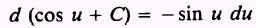andTherefore,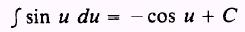Formula.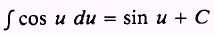PROOF: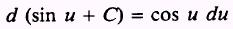Therefore,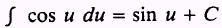Formula.PROOF: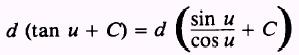and by the quotient rule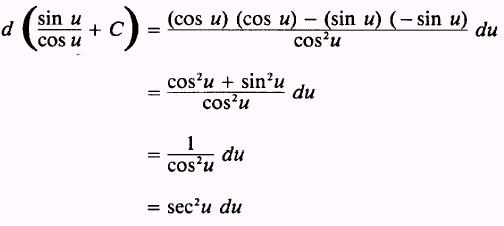Therefore,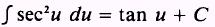To this point we have considered integrals of trigonometric functions that result in functions of the sine, cosine, and tangent. Those integrals that result in functions of the cotangent, secant, and cosecant are included in the following list of elementary integrals:EXAMPLE: Evaluate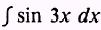SOLUTION: We need the integral in the form of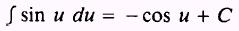We let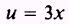so that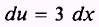but we do not have 3 dx. Therefore, we multiply the integral by 3/3 and rearrange as follows: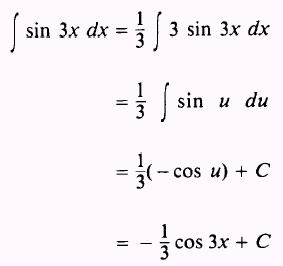EXAMPLE: EvaluateSOLUTION: Letso that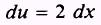Therefore,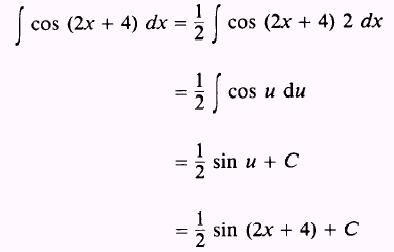EXAMPLE: Evaluate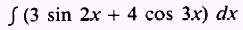SOLUTION: We use the rule for sums and write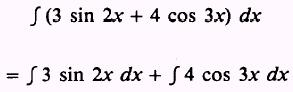Then, in the integrallet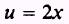so thatbut we have 3 dx Hence, proper compensation has to be made as follows:The second integralwith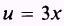and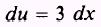is evaluated as follows: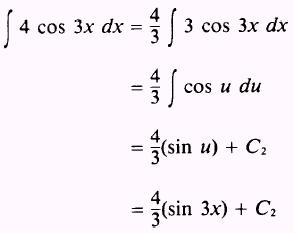Then, by combining the two solutions, we havewhere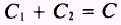EXAMPLE: Evaluate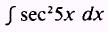SOL UTION: Letso that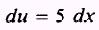We need 5 dx so we writeEXAMPLE: EvaluateSOL UTION: Let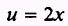so that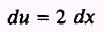We require du equal to 2 dx, so we write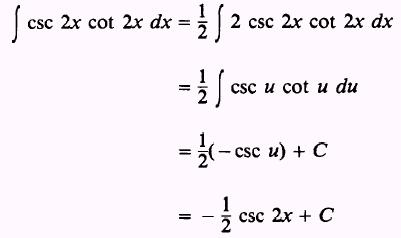EXAMPLE: Evaluate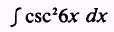SOL UTION: Let u=6x so thatthen,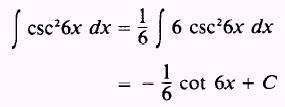EXAMPLE: EvaluateSOL UTION: Let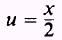so thatthen,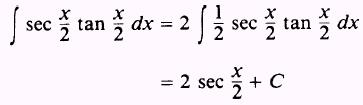Integrated Publishing, Inc. - A (SDVOSB) Service Disabled Veteran Owned Small Business